# ParallelR

Diving into Parallel Technology

0%

## Backgrounds

Deep Neural Network (DNN) has made a great progress in recent years in image recognition, natural language processing and automatic driving fields, such as Picture.1 shown from 2012  to 2015 DNN improved IMAGNET’s accuracy from ~80% to ~95%, which really beats traditional computer vision (CV) methods.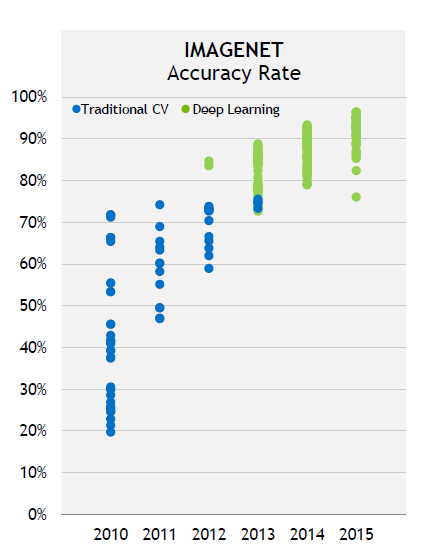Picture.1 - From NVIDIA CEO Jensen’s talk in CES16

In this post, we will focus on fully connected neural networks which are commonly called DNN in data science. The biggest advantage of DNN is to extract and learn features automatically by deep layers architecture, especially for these complex and high-dimensional data that feature engineers can’t capture easily, examples in Kaggle. Therefore, DNN is also very attractive to data scientists and there are lots of successful cases as well in classification, time series, and recommendation system, such as Nick’s post and credit scoring by DNN. In CRAN and R’s community, there are several popular and mature DNN packages including nnet, nerualnet, H2O, DARCH, deepnet and mxnet,  and I strong recommend H2O DNN algorithm and R interface.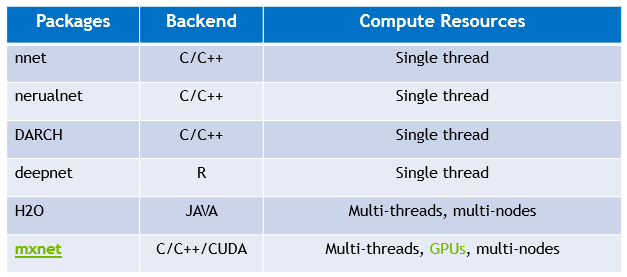So, why we need to build DNN from scratch at all? - Understand how neural network works Using existing DNN package, you only need one line R code for your DNN model in most of the time and there is an example by neuralnet. For the inexperienced user, however, the processing and results may be difficult to understand.  Therefore, it will be a valuable practice to implement your own network in order to understand more details from mechanism and computation views. - Build specified network with your new ideas DNN is one of rapidly developing area. Lots of novel works and research results are published in the top journals and Internet every week, and the users also have their specified neural network configuration to meet their problems such as different activation functions, loss functions, regularization, and connected graph. On the other hand, the existing packages are definitely behind the latest researches, and almost all existing packages are written in C/C++, Java so it’s not flexible to apply latest changes and your ideas into the packages. - Debug and visualize network and data As we mentioned, the existing DNN package is highly assembled and written by low-level languages so that it’s a nightmare to debug the network layer by layer or node by node. Even it’s not easy to visualize the results in each layer, monitor the data or weights changes during training, and show the discovered patterns in the network.

## Fundamental Concepts and Components

Fully connected neural network, called DNN in data science, is that adjacent network layers are fully connected to each other. Every neuron in the network is connected to every neuron in adjacent layers. A very simple and typical neural network is shown below with 1 input layer, 2 hidden layers, and 1 output layer. Mostly, when researchers talk about network’s architecture, it refers to the configuration of DNN, such as how many layers in the network, how many neurons in each layer, what kind of activation, loss function, and regularization are used.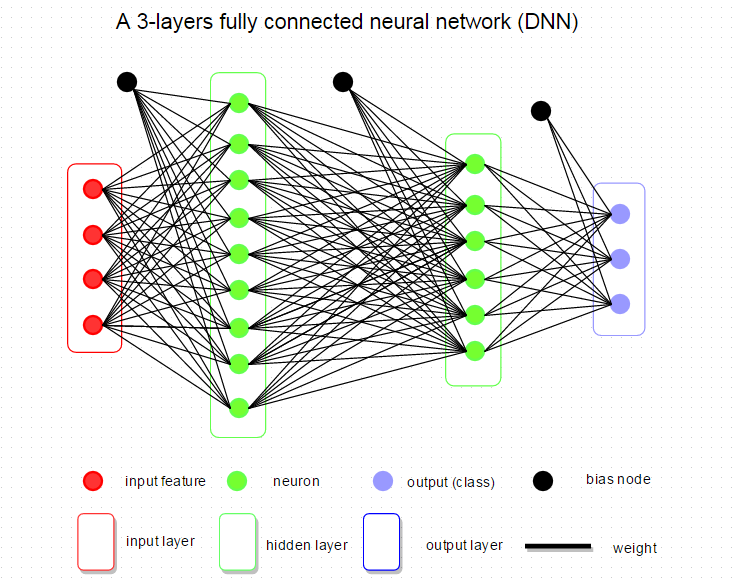Now, we will go through the basic components of DNN and show you how it is implemented in R. Weights and Bias Take above DNN architecture, for example, there are 3 groups of weights from the input layer to first hidden layer, first to second hidden layer and second hidden layer to output layer. Bias unit links to every hidden node and which affects the output scores, but without interacting with the actual data. In our R implementation, we represent weights and bias by the matrix. Weight size is defined by,

(number of neurons layer M) X (number of neurons in layer M+1)

and weights are initialized by random number from rnorm. Bias is just a one dimension matrix with the same size of  neurons and set to zero. Other initialization approaches, such as calibrating the variances with 1/sqrt(n) and sparse initialization, are introduced in weight initialization part of Stanford CS231n. Pseudo R code:

Another common implementation approach combines weights and bias together so that the dimension of input is N+1 which indicates N input features with 1 bias, as below code:

Neuron A neuron is a basic unit in the DNN which is biologically inspired model of the human neuron. A single neuron performs weight and input multiplication and addition (FMA), which is as same as the linear regression in data science, and then FMA’s result is passed to the activation function. The commonly used activation functions include sigmoid, ReLu, Tanh and Maxout. In this post, I will take the rectified linear unit (ReLU)  as activation function,  `f(x) = max(0, x)`. For other types of activation function, you can refer here.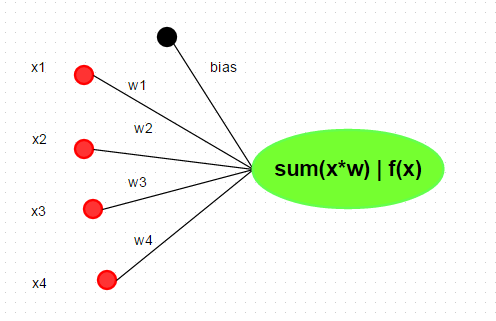In R, we can implement neuron by various methods, such as `sum(xi*wi)`. But, more efficient representation is by matrix multiplication. R code:

Implementation Tips _In practice, we always update all neurons in a layer with a batch of examples for performance consideration. Thus, the above code will not work correctly.

As below code shown,  `input %*% weights` and `bias` with different dimensions and  it can’t  be added directly. Two solutions are provided. The first one repeats bias_ ncol _times, however, it will waste lots of memory in big data input. Therefore, the second approach is better.

1. Element-wise max value for a matrix

Another trick in here is to replace `max` by `pmax` to get element-wise maximum value instead of a global one, and be careful of the order in `pmax` :)

Layer

• Input Layer

the input layer is relatively fixed with only 1 layer and the unit number is equivalent to the number of features in the input data.

• Hidden layers

Hidden layers are very various and it’s the core component in DNN. But in general,  more hidden layers are needed to capture desired patterns in case the problem is more complex (non-linear).

• Output Layer

The unit in output layer most commonly does not have an activation because it is usually taken to represent the class scores in classification and arbitrary real-valued numbers in regression. For classification, the number of output units matches the number of categories of prediction while there is only one output node for regression.

## Build Neural Network: Architecture, Prediction, and Training

Till now, we have covered the basic concepts of deep neural network and we are going to build a neural network now, which includes determining the network architecture, training network and then predict new data with the learned network. To make things simple, we use a small data set, Edgar Anderson’s Iris Data (iris) to do classification by DNN.

Network Architecture

IRIS is well-known built-in dataset in stock R for machine learning. So you can take a look at this dataset by the `summary` at the console directly as below.

R code:

From the summary, there are four features and three categories of Species. So we can design a DNN architecture as below.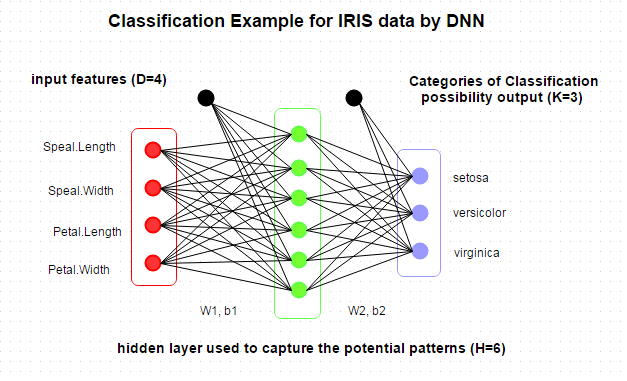And then we will keep our DNN model in a list, which can be used for retrain or prediction, as below. Actually, we can keep more interesting parameters in the model with great flexibility.

R code:

Prediction Prediction, also called classification or inference in machine learning field, is concise compared with training, which walks through the network layer by layer from input to output by matrix multiplication. In output layer, the activation function doesn’t need. And for classification, the probabilities will be calculated by softmax  while for regression the output represents the real value of predicted.   This process is called feed forward or feed propagation.

R code:

Training

Training is to search the optimization parameters (weights and bias) under the given network architecture and minimize the classification error or residuals.  This process includes two parts: feed forward and back propagation. Feed forward is going through the network with input data (as prediction parts) and then compute data loss in the output layer by loss function (cost function). “Data loss measures the compatibility between a prediction (e.g. the class scores in classification) and the ground truth label.” In our example code, we selected cross-entropy function to evaluate data loss, see detail in here. After getting data loss, we need to minimize the data loss by changing the weights and bias. The very popular method is to back-propagate the loss into every layers and neuron by gradient descent or  stochastic gradient descent which requires derivatives of data loss for each parameter (W1, W2, b1, b2). And back propagation will be different for different activation functions and see here and here for their derivatives formula and method, and Stanford CS231n for more training tips. In our example, the point-wise derivative for ReLu is: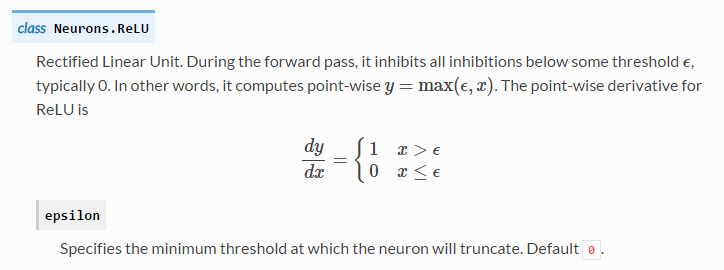R code:

## Testing and Visualization

We have built the simple 2-layers DNN model and now we can test our model. First, the dataset is split into two parts for training and testing, and then use the training set to train model while testing set to measure the generalization ability of our model.

R code

The data loss in train set and the accuracy in test as below: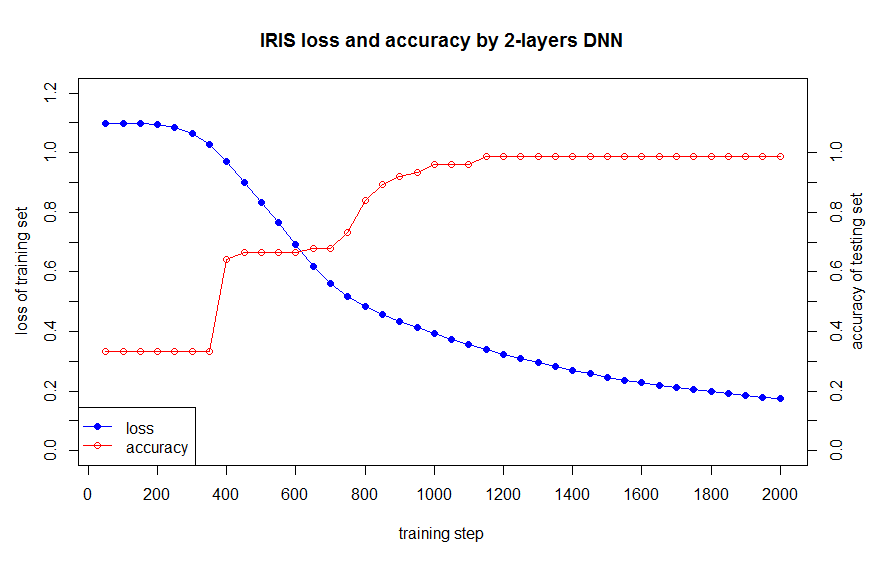Then we compare our DNN model with ‘nnet’ package as below codes.

Update: 4/28/2016:

Google’s tensorflow released a very cool website to visualize neural network in here. And we has taught almost all technologies as google’s website in this blog so you can build up with R as well :)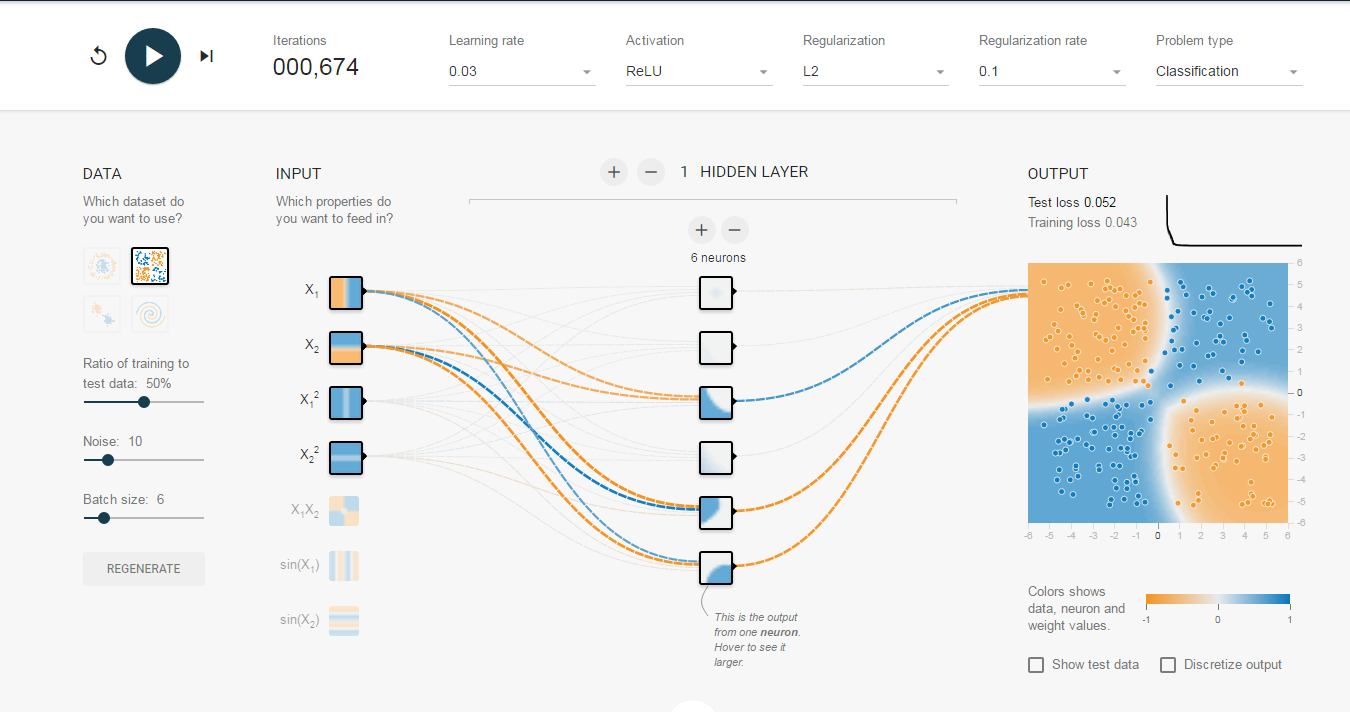## Summary

In this post, we have shown how to implement R neural network from scratch. But the code is only implemented the core concepts of DNN, and the reader can do further practices by:

• Solving other classification problem, such as a toy case in here
• Selecting various hidden layer size, activation function, loss function
• Extending single hidden layer network to multi-hidden layers
• Adjusting the network to resolve regression problems
• Visualizing the network architecture, weights, and bias by R, an example in here.

In the next post, I will introduce how to accelerate this code by multicores CPU and NVIDIA GPU.

Notes: 1. The entire source code of this post in here 2. The PDF version of this post in here 3. Pretty R syntax in this blog is Created by inside-R .org (acquired by MS and dead)